Courses

# Test: Building With Bricks

## 25 Questions MCQ Test Mathematics (Maths) for Class 4 | Test: Building With Bricks

Description
This mock test of Test: Building With Bricks for Class 4 helps you for every Class 4 entrance exam. This contains 25 Multiple Choice Questions for Class 4 Test: Building With Bricks (mcq) to study with solutions a complete question bank. The solved questions answers in this Test: Building With Bricks quiz give you a good mix of easy questions and tough questions. Class 4 students definitely take this Test: Building With Bricks exercise for a better result in the exam. You can find other Test: Building With Bricks extra questions, long questions & short questions for Class 4 on EduRev as well by searching above.
QUESTION: 1

### Where is the Jagriti school located

Solution:

Jagriti school is located in Murshidabad, West Bengal.

QUESTION: 2

### From where did Jamaal, Kaalu and Piyaar get the new idea for school building?

Solution:

Jamaal, Kaalu and Piyaar got the new idea for school building from Old Tomb of Murshid Kuli Khan

QUESTION: 3

### Old tomb of Murshid Kuli Khan is how many years old?

Solution:

Tomb of Murshid Kuli Khan is three hundred years old.

QUESTION: 4

Which of the following patterns are floor patterns?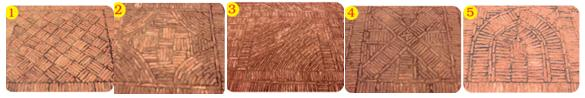Solution:

All the above are floor patterns.

QUESTION: 5

Which of the following pattern is circular?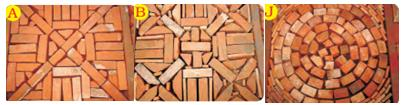Solution:

In ‘J’  all the bricks are placed in a small circle to form a large circle.

QUESTION: 6

In which of the following patterns, mirror halve can be seen?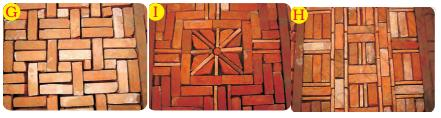Solution:

Dotted line in the pattern represents the line of symmetry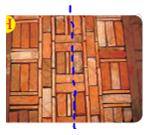QUESTION: 7

Which of the following shows three faces of a brick?

Solution:

Option (b) shows three faces of a brick.

QUESTION: 8

How many faces in all does a brick has?

Solution:

A brick has six faces- Top, Bottom and four sides.

QUESTION: 9

What is the shape of all six faces in a brick?

Solution:

All faces of the brick are Rectangle in shape.

QUESTION: 10

Which face of the brick is the smallest?

Solution:

If we see a brick from the top, its left and right-side faces are smaller as compared to the other faces.

QUESTION: 11

Which of these are the faces of a brick?

Solution:

All faces of the brick are rectangular in shape.

QUESTION: 12

Which of these is a drawing of a brick?

Solution:

All faces of the bricks are rectangular in shape.

QUESTION: 13

Which design of the wall is expected to be the weakest?

Solution:

Option b is expected to be the weakest because one brick is placed exactly one above the other.So the stability of the wall would be lesser than other options.

QUESTION: 14

Below pattern of wall design is matching with which brick wall.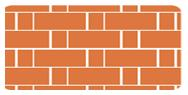Solution:

Long side and small side of the bricks are matching with option c.

QUESTION: 15

How many different patterns can you see in these two photos?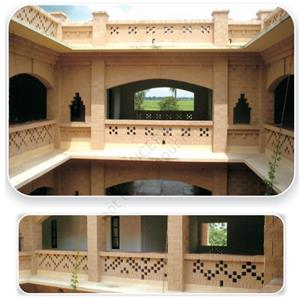Solution:

here are five types of Jaali design shown in the two pictures.

QUESTION: 16

Which shape can be made by placing a brick in the garden?

Solution:

We can keep the brick in the garden as shown in picture below.It makes a triangle shape.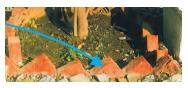QUESTION: 17

What type of design you can see in the below picture.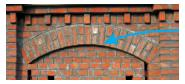Solution:

An arch type design is shown in the picture.

QUESTION: 18

Burnt bricks can be further classified into how many types?

Solution:

There can be 4 sub-divisions of burnt bricks. They are first class, second class, third class and kiln rejected bricks.

QUESTION: 19

In the picture below,how many faces of the longest brick can be seen from the top?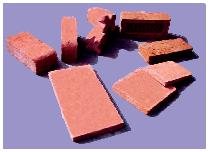Solution:

As it is the top view of the longest brick, only one face can be seen.

QUESTION: 20

Which of the following is used to make a brick?

Solution:

Clay and soil are used to make a brick.

QUESTION: 21

If a brick length is 10 centimeters, then how many bricks need to put in a straight line to make a wall of one-meter length.

Solution:

One meter is equal to hundred centimeters. If we divide 100/10= 10, so 10 bricks are required.

QUESTION: 22

Arrange the following images related to brick making in correct order.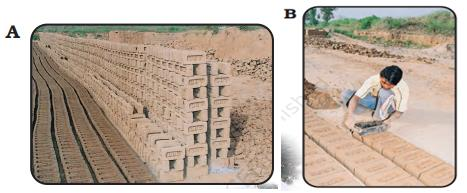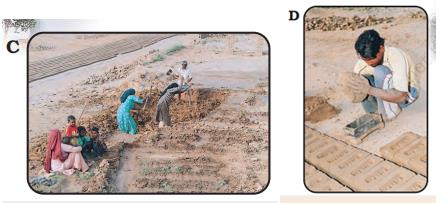Solution:

Steps followed in making bricks are collection of clay, pouring in mold, removing from mold and drying.

QUESTION: 23

Which is the correct numeral representation of one hundred thousand?

Solution:

One hundred thousand is equal to one lakh.

QUESTION: 24

Bhajan went to buy bricks. The price was given for one thousand bricks. The prices were also different for different types of bricks. Bhajan decided to buy the new bricks from Brickabad. He bought three thousand bricks. How much did he pay?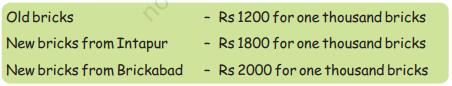Solution:

Price of Thousand bricks = 2000 Rs
Price of One brick = 2000/1000 = 2 Rs
Price of Three thousand bricks= 2X3000 =6000 Rs
So, Bhajan needs to pay 6000 Rs for three thousand bricks.

QUESTION: 25

Bhajan went to buy bricks. The price was given for one thousand bricks. The prices were also different for different types of bricks. Bhajan decided to buy the old bricks. He bought five hundred bricks. How much did he pay?Solution:

Thousand bricks’ price for old bricks = 1200 Rs

One brick’s price  = 1200/1000 = 1.2 Rs

Five hundred bricks’ price  = 1.2X500 =600 Rs

So, Bhajan needs to pay 600 Rs for 500 old bricks.